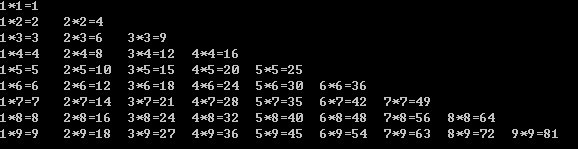# 从零开始学编程---第一步-C语言（十二）

break不仅能跳出switch语句，也能跳出循环语句

#include <stdio.h>
main()
{
int i;
for(i=1;i<=10;i++)
{
printf("%d ",i);
}

}

#include <stdio.h>
main()
{
int i;
for(i=1;i<=10;i++)
{
printf("%d ",i);
if(i==5)
{break;}
}

}

#include <stdio.h>
main()
{
int i=1;
for(;;)
{
printf("%d ",i);
i++;
if(i>10)
{break;}
}

}

continue是继续的意思
break是循环提前跳出，与之相反，continue就是强迫循环提前继续
continue也需要和if在一起

continue;

continue强迫循环提前继续是个概念？

#include <stdio.h>
main()
{
int i;
for(i=1;i<=10;i++)
{
if(i==5)
{

printf("找到了五 ");

}
printf("%d ",i);

}

}

#include <stdio.h>
main()
{
int i;
for(i=1;i<=10;i++)
{
if(i==5)
{

printf("找到了五 ");
continue;
}
printf("%d ",i);

}

}

continue的作用你应该明白了吧

continue的功能就是强迫循环进入新的循环周期

break和continue也能在while语句中使用

#include <stdio.h>
main()
{
int i;
for(i=1;i<=10;i++)
{
if(i==5)
{

printf("找到了五 ");
continue;
}
if(i==9)
{
printf("找到了九");
break;
}

printf("%d ",i);

}

}

break和continue的具体用处大家会在以后程序中看到

for,while,do-while可以相互包含，构成多重循环

1)for(){…
while()
{…}
…
}
(2)do{
…
for()
{…}
…
}while();
(3)while(){
…
for()
{…}
…
}
(4)for(){
…
for(){
…
}
}x。y都需要每循环一次递增1

1*1=1

1*2=2 2*2=4

1*3=3 2*3=6 3*3=9

。。。。。。

x的循环条件就是while(x<=y)

。。。。。。。。。。。。。

x每次循环开始都是从1开始，所以每次都需要初始化x为1

y每次循环一次    x初始化1 再X自己循环从1到y循环的次数

#include <stdio.h>
main()
{
int x,y;
y=1;
while(y<=9)
{
x=1;
while(x<=y)
{
printf("%d*%d=%d\t",x,y,x*y);
x++;
}
printf("\n");
y++;
}

}

while(x<=y)
{
printf("%d*%d=%d\t",x,y,x*y);
x++;
}

%4d的含义我以前说过了，这里在说一次，%d 前面加数字，例如%4d 表示按4位数输出，%d如果多于4位数，按4位输出，少于4位 按原样输出且靠右 即右对齐

#include <stdio.h>
main()
{
int x,y;
y=1;
do
{
x=1;
do

{
printf("%d*%d=%d\t",x,y,x*y);
x++;
}while(x<=y);
printf("\n");
y++;

}
while(y<=9);

}

#include <stdio.h>
main()
{
int x,y;    //x表示列，y表示行
for(y=1;y<=9;y++) 每循环一次回车
{
for(x=1;x<=y;x++) 从1到y循环打印x*y=x*y
{
printf("%d*%d=%d\t",x,y,x*y);
}
printf("\n");
}

}

#include <stdio.h>
main()
{
int x,y;
for(y=9;y>=1;y--)
{
for(x=1;x<=y;x++)
{
printf("%d*%d=%d\t",x,y,x*y);
}
printf("\n");
}

}

(8)
100%

(0)
0%